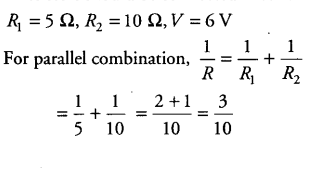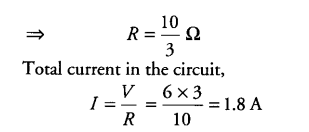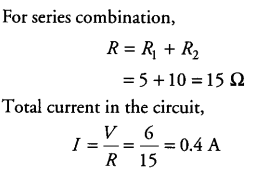# Two resistors with resistances 5 \$\Omega \$ and 10 \$\Omega \$ respectively

Two resistors with resistances 5 \$\Omega \$ and 10 \$\Omega \$ respectively are to be connected to a battery of emf 6 V so as to obtain
(i) (a) minimum current.
(b) maximum current.
(ii) How will you connect the resistances in each case?
(iii) Calculate the strength of the total current in the circuit in the two cases.

(i) (a) For obtaining minimum current, the two resistors should be connected in parallel.
(b) For obtaining maximum current, the two resistors should be connected in series.

(ii)(iii)